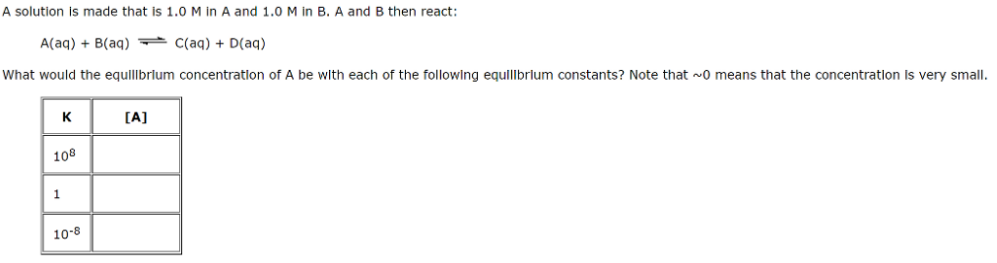# Problem: A solution is made that is 1.0 M in A and 1.0 M in B, A and B then react: A(aq) + B(aq) ⇌ C(aq) + D(aq) What would the equilibrium concentration of A be with each of the following equilibrium constants? Note that ~0 means that the concentration is very small.

###### FREE Expert Solution
85% (416 ratings)###### Problem Details

A solution is made that is 1.0 M in A and 1.0 M in B, A and B then react:

A(aq) + B(aq) ⇌ C(aq) + D(aq)

What would the equilibrium concentration of A be with each of the following equilibrium constants? Note that ~0 means that the concentration is very small.